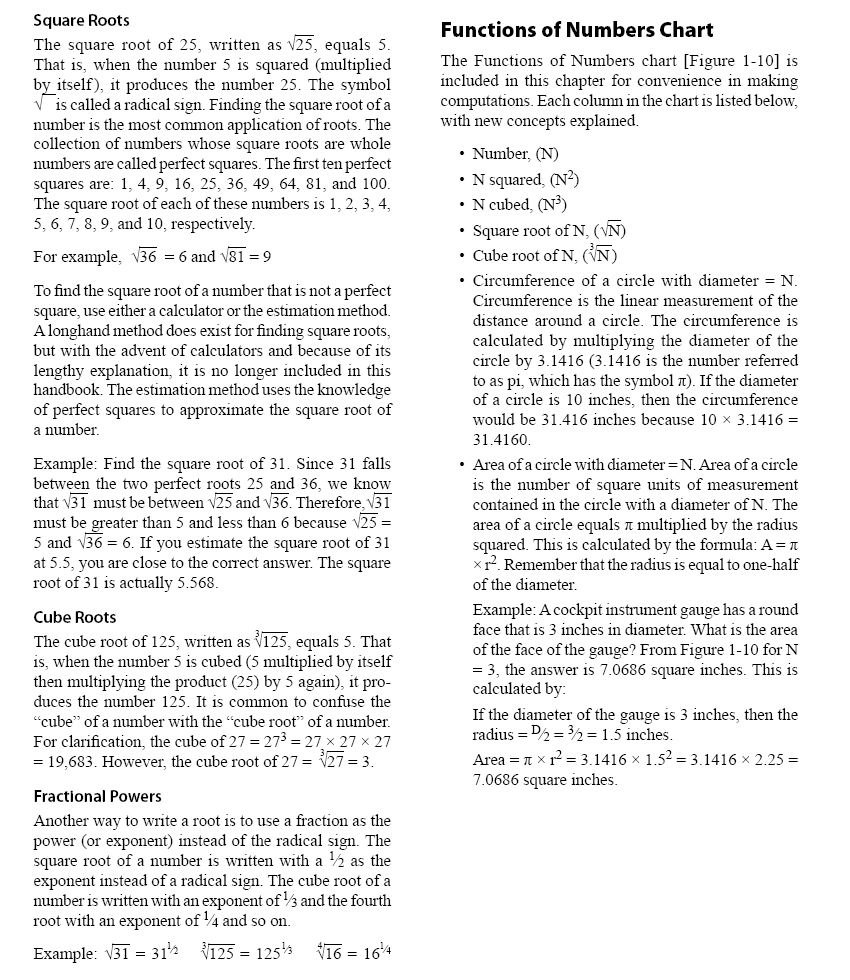Scientific Notation Scientific notation is used as a type of shorthand to express very large or very small numbers. It is a way to write numbers so that they do not take up as much space on the page. The format of a number written in scientific notation has two parts. The first part is a number greater than or equal to 1 and less than 10 (for example, 2.35). The second part is a power of 10 (for example, 106). The number 2,350,000 is expressed in scientific notation as 2.35 x 106. It is important that the decimal point is always placed to the right of the first digit. Notice that very large numbers always have a positive power of 10 and very small numbers always have a negative power of 10. Example: The velocity of the speed of light is over 186,000,000 mph. This can be expressed as 1.86 x 108 mph in scientific notation. The mass of an electron is approximately 0.000,000,000,000,000,000,000,000 ,000,911 grams. This can be expressed in scientific notation as 9.11 x 10-28 grams.
 ©AvStop Online Magazine                                                                                                                                                      Contact Us              Return To Books